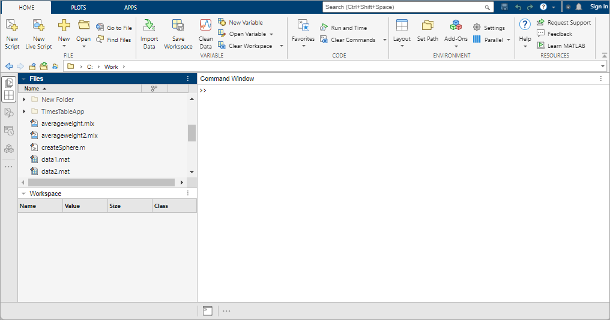## Desktop Basics

When you start MATLAB®, the desktop appears in its default layout.The desktop includes these panels:

• Current Folder — Access your files.

• Command Window — Enter commands at the command line, indicated by the prompt (`>>`).

• Workspace — Explore data that you create or import from files.

As you work in MATLAB, you issue commands that create variables and call functions. For example, create a variable named `a` by typing this statement at the command line:

`a = 1`

MATLAB adds variable `a` to the workspace and displays the result in the Command Window.

```a = 1```

Create a few more variables.

```b = 2 ```
```b = 2```
`c = a + b`
```c = 3```
`d = cos(a)`
```d = 0.5403```

When you do not specify an output variable, MATLAB uses the variable `ans`, short for answer, to store the results of your calculation.

`sin(a)`
```ans = 0.8415```

If you end a statement with a semicolon, MATLAB performs the computation, but suppresses the display of output in the Command Window.

`e = a*b;`

You can recall previous commands by pressing the up- and down-arrow keys, ↑ and ↓. Press the arrow keys either at an empty command line or after you type the first few characters of a command. For example, to recall the command `b = 2`, type `b`, and then press the up-arrow key.